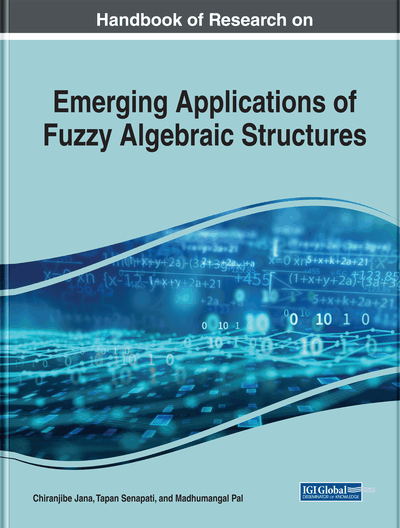Uni-Hesitant Fuzzy Set Approach to the Ideal Theory of BCK-Algebras

G. Muhiuddin (University of Tabuk, Saudi Arabia)
DOI: 10.4018/978-1-7998-0190-0.ch008
Available
\$37.50
No Current Special Offers

Abstract

In this chapter, the author studies the uni-hesitant fuzzy set-theoretical approach to the ideals of BCK-algebras. For a hesitant fuzzy set H on S and a subset of [0,1], the set L(H;ʎ):={x∈S|xH⊆ʎ}, is called the uni-hesitant level set of H. Moreover, the author discusses the relations between uni-hesitant fuzzy commutative ideals and uni-hesitant fuzzy ideals. Further, he considered the characterizations of uni-hesitant fuzzy commutative ideals in BCK-algebras. Finally, he proved some conditions for a uni-hesitant fuzzy ideal to be a uni-hesitant fuzzy commutative ideal.
Chapter Preview
Top

2 Preliminaries

A an extensively investigated by several researchers.

An algebra (X;*,0) of type (2,0) is called a BCI-algebra if it satisfies the following conditions:

• (∀x,y,zX) (((x*y)*(x*z))*(z*y)=0),

• (∀x,yX) ((x*(x*y))*y=0),

• (∀xX) (x*x=0),

• (∀x,yX) (x*y=0, y*x=0 ⇒ x=y).

If a BCI-algebra X satisfies the following identity:

• (∀xX) (0*x=0),

then X is called a BCK-algebra. Any BCK/BCI-algebra X satisfies the following axioms:
• (∀xX) (x*0=x),

• (∀x,y,zX) (xyx*zy*z, z*yz*x),

• (∀x,y,zX) ((x*y)*z=(x*z)*y),

• (∀x,y,zX) ((x*z)*(y*z)≤x*y)

where xy if and only if x*y=0 A BCK-algebra X is s said to be commutative if xy=yx for all x,yX where xy=y*(y*x). A nonempty subset S of a BCK/BCI-algebra X is called a subalgebra of X if x*yS for all x,yS. A subset A of a BCK/BCI-algebra X is called an ideal of X if it satisfies:

Complete Chapter List

Search this Book:
Reset